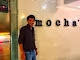Bangalore, India
I am an Oracle Certified Professional working in SAP Labs. Everything written on my blog has been tested on my local environment, Please test before implementing or running in production. You can contact me at amit.rath0708@gmail.com.

## Tuesday, September 24, 2013

### DB Time in AWR report

DB Time in AWR report is the total time for which all user process are either working or waiting in Database. In other words , DB Time is the time spent in database by all user processes including CPU time, I/O time and Wait time and excludes idle time for a particular Snap Interval.

When We generate AWR report for a particular time interval. DB time can be less than AWR generation time interval or greater than. If DB time is too high as compared to AWR generation time interval then heavy activity is going on database and if less, then database is in idle state for most of the time.

Let suppose AWR is generated for 2 hours, then AWR generation time interval  is 120 mins. Every user process in Oracle database is calculated on the basis of DB Time in AWR report. For Ex:-

 Begin Snap: 34436 03-Sep-13 16:00:46 420 23.2 End Snap: 34478 05-Sep-13 10:00:38 360 25.8 Elapsed: 2,519.88 (mins) DB Time: 22,362.47 (mins)

Here DB time is too high as compared to Elapsed time means heavy activity is going on database.

Statistic NameTime (s)% of DB Time
sql execute elapsed time 862,534.11 64.28
DB CPU 573,949.72 42.78
connection management call elapsed time 16,980.05 1.27
parse time elapsed 8,017.36 0.60

Above are statistics consumes DB time , This % can be greater than 100 % . If sql execute elapsed time is having a greater value means your database spends most of its time in executing SQL's.

As a DBA we need to regularly look into AWR reports so that we are aware that every thing is working fine. But for Production databases we can't generate AWR's for every Snap interval. PFB below query which will give the snap time for which DB time is too high and then we can generate AWR report for that Snap period and analyze the things.

Query to generate DB time of all Snap Intervals in a week  :-

Standalone Database :-

col begin_interval_time_N format A35 trunc
col end_interval_time_N format A35 trunc
col HOST_NAME format A20 trunc
col INSTANCE_NUMBER format 9 heading “I”
col begin_interval_time_N
col end_interval_time_N
select  A.SNAP_ID , A.INSTANCE_NUMBER , C.HOST_NAME  , MIN(A.begin_interval_time) OVER (partition by A.dbid, A.snap_id) AS begin_interval_time_N
, MIN(A.end_interval_time) OVER (partition by A.dbid, A.snap_id) AS end_interval_time_N  , (B.VALUE - LAG(B.VALUE,1,0) OVER
(ORDER BY A.SNAP_ID))/1000000/60 as DB_TIME  from dba_hist_snapshot A , DBA_HIST_SYS_TIME_MODEL B,dba_hist_database_instance C
where A.SNAP_ID=B.SNAP_ID  and A.DBID=B.DBID  And A.INSTANCE_NUMBER=B.INSTANCE_NUMBER  and B.STAT_NAME Like 'DB time'
and A.DBID=C.DBID  and A.INSTANCE_NUMBER=C.INSTANCE_NUMBER  and A.startup_time = C.startup_time  and
A.begin_interval_time >= trunc(sysdate) - 1 and A.begin_interval_time <= trunc(sysdate) ;

RAC Database , We have to generate instance wise :-

col begin_interval_time_N format A35 trunc
col end_interval_time_N format A35 trunc
col HOST_NAME format A20 trunc
col INSTANCE_NUMBER format 9 heading “I”
col begin_interval_time_N
col end_interval_time_N
select  A.SNAP_ID , A.INSTANCE_NUMBER , C.HOST_NAME  , MIN(A.begin_interval_time) OVER (partition by A.dbid, A.snap_id) AS begin_interval_time_N
, MIN(A.end_interval_time) OVER (partition by A.dbid, A.snap_id) AS end_interval_time_N  , (B.VALUE - LAG(B.VALUE,1,0) OVER
(ORDER BY A.SNAP_ID))/1000000/60 as DB_TIME  from dba_hist_snapshot A , DBA_HIST_SYS_TIME_MODEL B,dba_hist_database_instance C
where A.SNAP_ID=B.SNAP_ID  and A.DBID=B.DBID  And A.INSTANCE_NUMBER=B.INSTANCE_NUMBER  and B.STAT_NAME Like 'DB time'   and A.DBID=C.DBID  and A.INSTANCE_NUMBER=C.INSTANCE_NUMBER  and A.startup_time = C.startup_time  and
A.begin_interval_time >= trunc(sysdate) - 1 and A.begin_interval_time <= trunc(sysdate) and A.INSTANCE_NUMBER=1;

Regards,
Amit Rath

1.1.